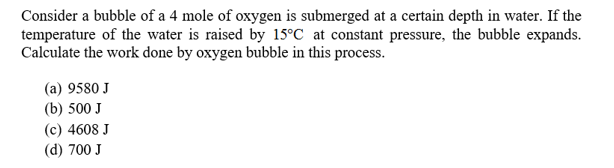# Consider a bubble of a 4 mole of oxygen is submerged at a certain depth in water. If thetemperature of the water is raised by 15°C at constant pressure, the bubble expands.Calculate the work done by oxygen bubble in this process.(a) 9580 J(b) 500 J(c) 4608 J(d) 700 J

Question
227 viewshelp_outlineImage TranscriptioncloseConsider a bubble of a 4 mole of oxygen is submerged at a certain depth in water. If the temperature of the water is raised by 15°C at constant pressure, the bubble expands. Calculate the work done by oxygen bubble in this process. (a) 9580 J (b) 500 J (c) 4608 J (d) 700 J fullscreen
check_circle

Step 1

Consider the temperature of bubble at the constant pressure P be T1 when the volume is V1, the temperature of bubble at the constant pressure P be T2 when the volume is V2, on the X scale be t.

The change in temperature is,

Step 2

Write the ideal gas equation for the initial temperature and volume of the bubble.

Step 3

Write the ideal gas equation for the final t...

### Want to see the full answer?

See Solution

#### Want to see this answer and more?

Solutions are written by subject experts who are available 24/7. Questions are typically answered within 1 hour.*

See Solution
*Response times may vary by subject and question.
Tagged in

### Thermodynamics